The International Information Center for Structural Engineers

Wednesday, 23 September 2015 00:00cat

## Calculation Example: Shear force On A Column

Written by  TheStructuralEngineer.info

A force is acting at the top of a building frame as shown. The supporting columns are of equal height and are fixed at the base. The modulus of elasticity E is the same for each column. Assuming the top plate is rigid and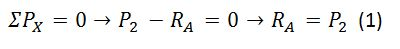What is the shear distributed to the first column?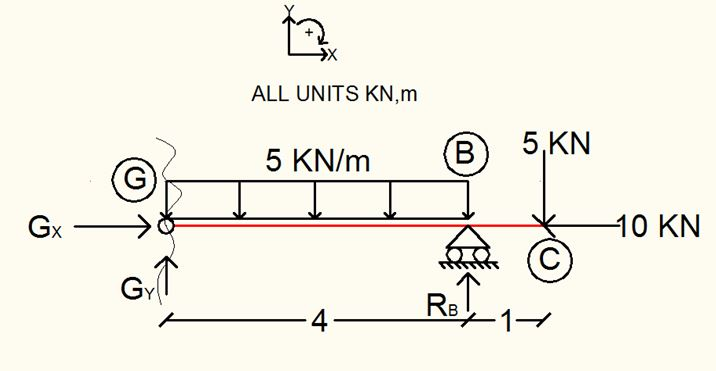Since the columns are rigid, the force is distributed to the columns in proportion to their rigidities (or stiffnesses). The composite stiffness is the sum of the individual column stiffnesses.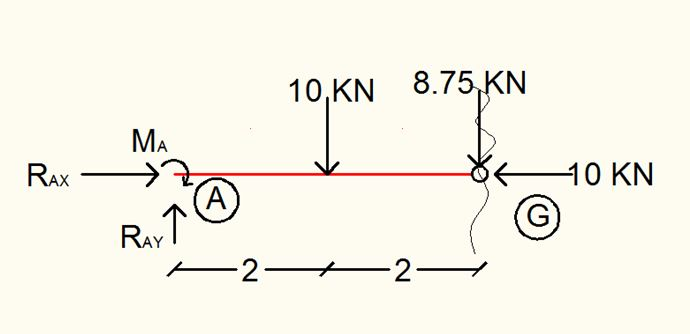Substitute for K1, K2 and K3. Then simplify.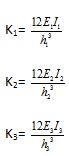It is given that E1= E2 =E3 and h1=h2=h3. Therefore,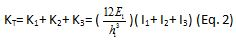Substitute Eq. 2 into Eq. 1 and solve.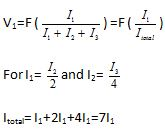Therefore,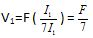• Default
• Title
• Date
• Random
• ## Keller lays foundation for Aston Martin tower

Keller business units HJ Foundation and Hayward Baker worked on the foundation system of one of Miami's most prestigious, eye-catching